JEE  >  Integer Answer Type Questions: Properties of triangle

# Integer Answer Type Questions: Properties of triangle - Notes | Study All Types of Questions for JEE - JEE

 1 Crore+ students have signed up on EduRev. Have you?

Q.1. If in a triangle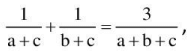then angle C is π/k, the numerical quantity k must be

Ans. 3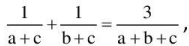will simplify to
a2 + b2- c2 = ab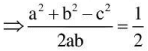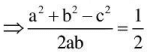⇒ cos C = 1/2 ⇒ c = 60o

Q.2. If b + c = 3a, then cot B/2 cot C/2 must be equal to

Ans. 2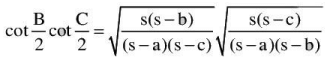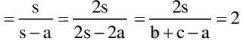Q.3. If in a ΔABC, a/cosA = b/cosB, then k sin A cos B = sin C. Where k is equal to . .

Ans. 2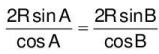or sin A cos B = cos A sin B
or 2sin A cos B = cos A sin B + sin A cosB = sin (A + B) = sin C

Q.4. In a triangle ABC, side AB = 20, AC = 11, BC = 13, then the diameter of the semicircle inscribed in triangle ABC, whose diameter lies on AB and is touching AC and BC is ___

Ans. 11
CT1 = CT2 = x
AB = 20
AC = 11
BC = 13
In ΔABC sinA = 3/5 and sinB = 33/65 also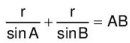⇒ r = 11/2

Q.5. A cyclic quadrilateral ABCD of area 3√3/4 is inscribed in a unit circle. If one of its sides AB = 1 and the diagonal BD = √3 then find the sum of all its sides.

Ans. 4
By sine rule AB = 1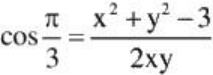4.

The document Integer Answer Type Questions: Properties of triangle - Notes | Study All Types of Questions for JEE - JEE is a part of the JEE Course All Types of Questions for JEE.
All you need of JEE at this link: JEE

## All Types of Questions for JEE

376 docs
 Use Code STAYHOME200 and get INR 200 additional OFF

## All Types of Questions for JEE

376 docs

Track your progress, build streaks, highlight & save important lessons and more!

,

,

,

,

,

,

,

,

,

,

,

,

,

,

,

,

,

,

,

,

,

;